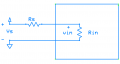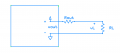# [Amplifiers] Calculating source-to-load voltage gain

#### nDever

Joined Jan 13, 2011
153
Hi guys,

I'm doing some elementary amplifier exercises, and decided to do this one.An amplifier has a specified voltage gain of 100, input resistance 100k$$\Omega$$, and output resistance 10$$\Omega$$. Calculate the source-to-load voltage gain for a source resistance of 10k$$\Omega$$ and a load resistance 100$$\Omega$$.

My answer comes out to be the reciprocal of the correct answer; clearly, I'm going wrong somewhere.
____________________________________________

Work:

$$A_v=100$$
$$R_{in}=100k\Omega$$
$$R_{out}=10\Omega$$
$$R_{s}=10k\Omega$$
$$R_{L}=100\Omega$$
____________________________________________

$$v_{in}=\frac{R_{in}v_{s}}{R_{in}+R_{s}}=\frac{100k{\Omega}v_{s}}{110k\Omega}=0.91v_{s}$$,

so

$$v_{s}=1.1v_{in}$$
____________________________________________

$$v_{L}=\frac{R_{L}v_{out}}{R_{L}+R_{out}}=\frac{100{\Omega}v_{out}}{110\Omega}=0.91v_{out}$$
____________________________________________

$$\frac{v_{s}}{v_{L}}=\frac{1.1v_{in}}{0.91v_{out}}=\frac{1.1}{0.91}*\frac{1}{100}=\frac{1.1}{91}=0.0121$$

Last edited:

#### Veracohr

Joined Jan 3, 2011
765
Voltage gain is expressed as the ratio of the load/output voltage to the source/input voltage: Vl/Vs.

You have Vs/Vl.